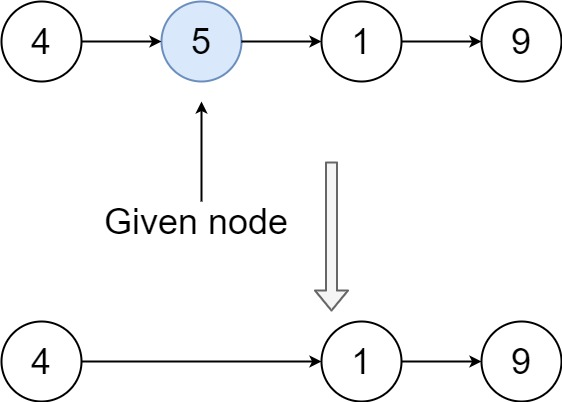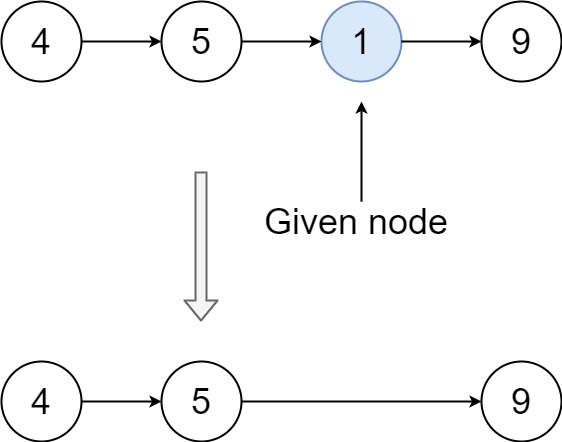0237. Delete Node in a Linked List

# 237. Delete Node in a Linked List#

## 题目 #

Write a function to delete a node in a singly-linked list. You will not be given access to the `head` of the list, instead you will be given access to the node to be deleted directly.

It is guaranteed that the node to be deleted is not a tail node in the list.

Example 1:``````Input: head = [4,5,1,9], node = 5
Output: [4,1,9]
Explanation:You are given the second node with value 5, the linked list should become 4 -> 1 -> 9 after calling your function.

``````

Example 2:``````Input: head = [4,5,1,9], node = 1
Output: [4,5,9]
Explanation:You are given the third node with value 1, the linked list should become 4 -> 5 -> 9 after calling your function.

``````

Example 3:

``````Input: head = [1,2,3,4], node = 3
Output: [1,2,4]

``````

Example 4:

``````Input: head = [0,1], node = 0
Output: 

``````

Example 5:

``````Input: head = [-3,5,-99], node = -3
Output: [5,-99]

``````

Constraints:

• The number of the nodes in the given list is in the range `[2, 1000]`.
• `1000 <= Node.val <= 1000`
• The value of each node in the list is unique.
• The `node` to be deleted is in the list and is not a tail node

## 代码 #

``````package leetcode

import (
"github.com/halfrost/leetcode-go/structures"
)

// ListNode define
type ListNode = structures.ListNode

/**
* type ListNode struct {
*     Val int
*     Next *ListNode
* }
*/
func deleteNode(node *ListNode) {
node.Val = node.Next.Val
node.Next = node.Next.Next
}
``````Apr 8, 2023Edit this page